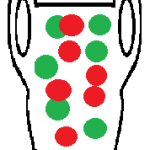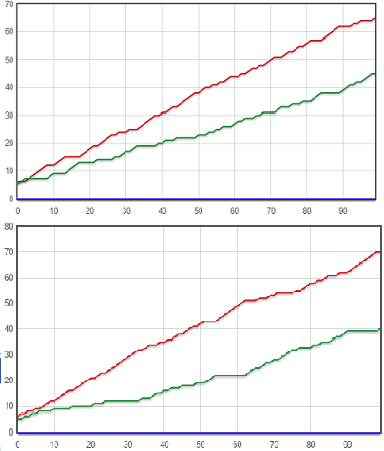# Pólya Urn: Definition, Examples

Urn models > Pólya Urn

## What is a Pólya Urn?Pólya’s urn is a famous sampling model used in probability. Let’s say you had an urn with red and green balls. You choose one ball at random, note the color, and replace the ball in the urn along with another ball of the same color. The resulting model is called Polya’s urn process.

In most cases, the process is used only with non-negative integers so that the process can continue indefinitely.

## Interesting Probabilities

At any time, the number of balls in the urn is t + red balls + green balls, where t is the number of trials. At any time, the odd of picking a red ball (or a green ball) are exactly the same. That is, for any time t≥1, the probability of picking a red ball is:
PRt = r / (r + g)
…and the probability of picking a green ball is:
PGt = g / (r + g).

If you actually run a simulation (you can find one here on Andrew Mauboussin’s website), you might be surprised at the results. Faulty logic (similar to the Gambler’s Fallacy) might tell you that if you start off with an equal ratio of colored balls, you’re going to end up with an equal ratio of colored balls. However, that isn’t true.

Let’s say you start off with equal numbers of balls (5 red, 5 green). After the first trial, if you choose a red ball, there will be 6 red and 5 green balls in the jar. That creates an automatic disparity, where you’ll be more likely to choose a red ball than a green ball in the next trial. The odds are now 6/11 that you’ll choose a red ball and 5/11 that you’ll choose green. This factor can snowball out of proportion. In fact, there are endless ways the disparity could play out. For 100 trials, here are two ways the simulation plays out:## Uses

The Polya Urn is used as an exercise in probability, much in the same way basic probability is introduced using numbers drawn from a bingo machine or cards drawn from a deck. The urn shows how small imbalances (like one extra red ball) can be magnified over time. Although interesting to study for theory purposes, it’s too simple a model to have any use in real-life situations, where multiple factors tend to affect outcomes.

## References

Mahmoud, A. (2008). Polya Urn Models. Chapman & Hall/CRC.

CITE THIS AS:
Stephanie Glen. "Pólya Urn: Definition, Examples" From StatisticsHowTo.com: Elementary Statistics for the rest of us! https://www.statisticshowto.com/polya-urn/